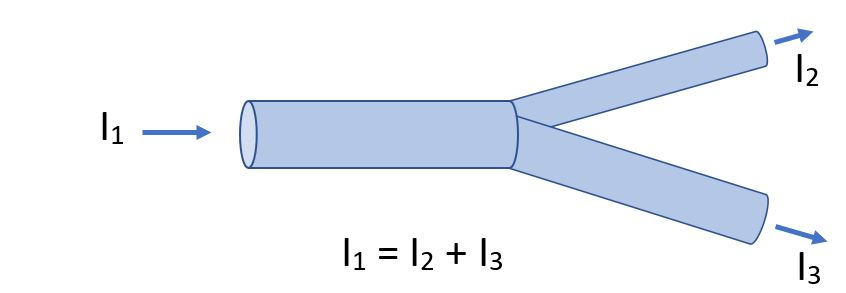﻿ Current and resistance

# Electric current

## Flow of electrons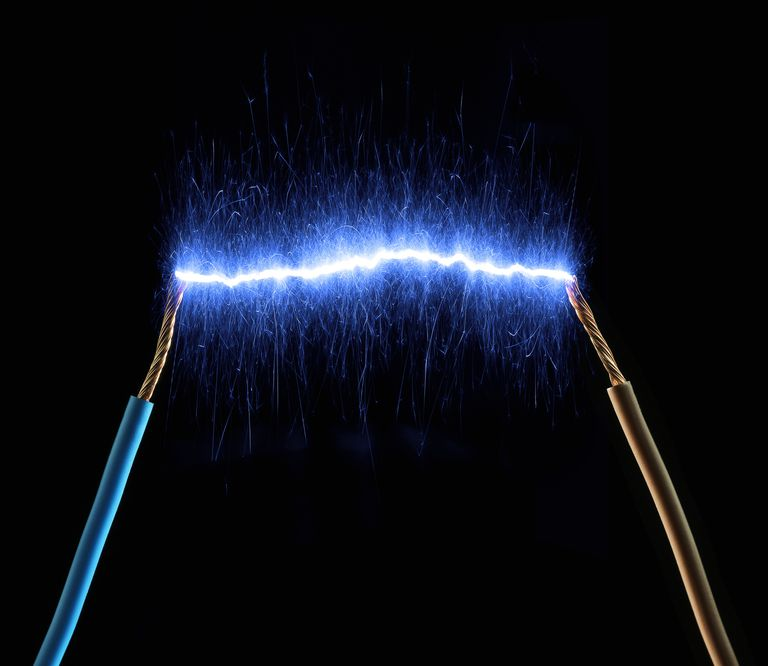### Air is typically a poor conductor, but with a high enough potential difference, electricity can flow through air.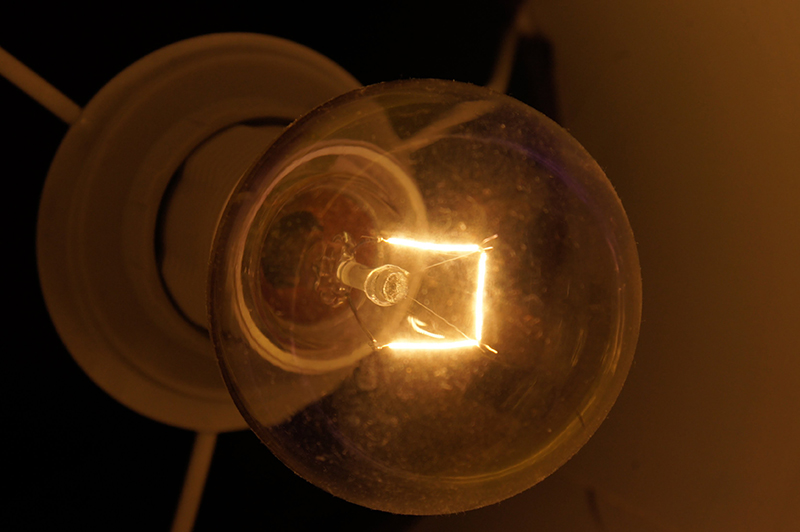### Electric current is what makes the filament of a light bulb glow.### Water current is simply how much water passes per second. Electrical current is similar; in this case, it is the flow of electric charges, measured in coloumbs per second.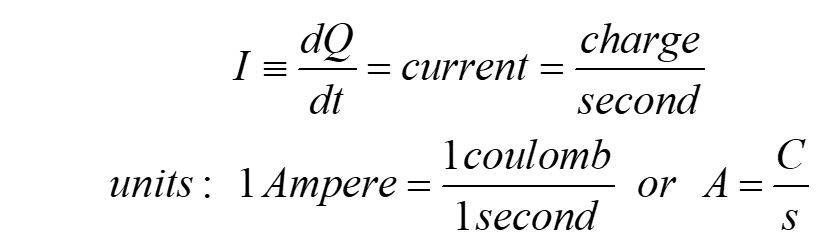## Drift speed

### The electromagnetic signal moves at the speed of light, but the electrons move much more slowly. We define the drift speed as the speed that the electrons move through the wire.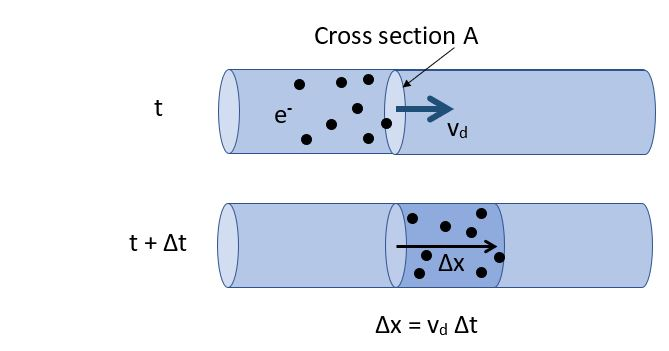### This diagram depicts electrons moving through a wire of cross-section A, from a time span from t to t + Δt. The electrons undergo collisions and have some random motion, but move as a group at drift speed vd.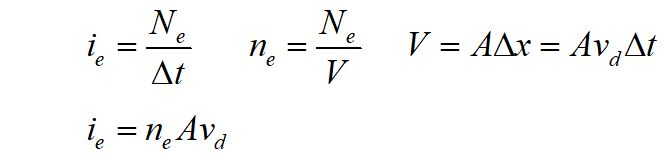### We define the electron current as the number of electrons per time passing a cross-section. We can also write the electron current in terms of the number density (electrons per volume) and the drift speed.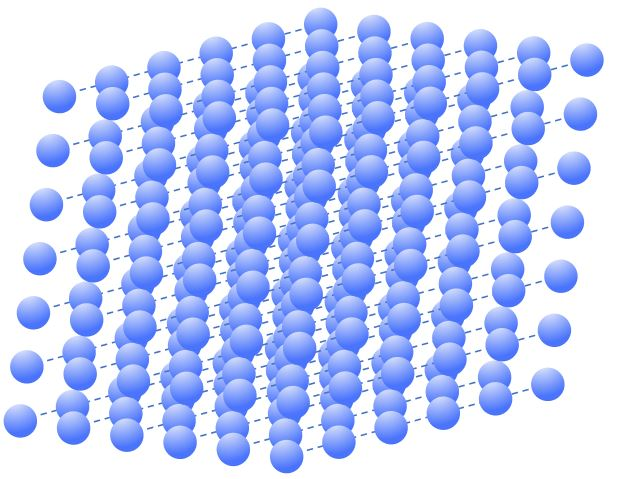### Recall our model of a conductor as a lattice of atoms, nuclei with electron shells. These atoms are ions, since the valence electrons are free to move within the lattice. The potential difference from a battery or charged capacitor sets up a nonuniform charge distribution. When the circuit is completed, the electrons have a pathway to flow, and move through the lattice.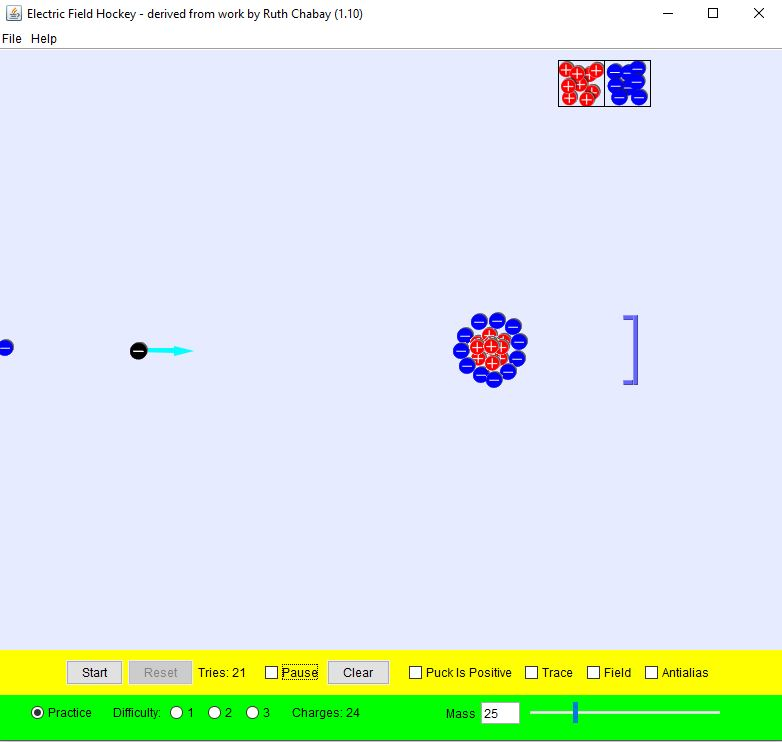### While moving through the lattice, electrons undergo collisions with the ions in the metal. Valence electrons are free to move within the lattice. We can model a collision using this PHeT simulation.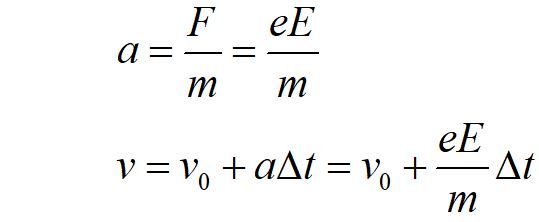The electric field provides the force on the electrons to speed them up between collisions. Recall that the electric force can be defined as F = qE. Here we define the charge of an electron as e, to write F = eE. We can use kinematics to write the equation of motion for electrons under the acceleration provided by the electric field.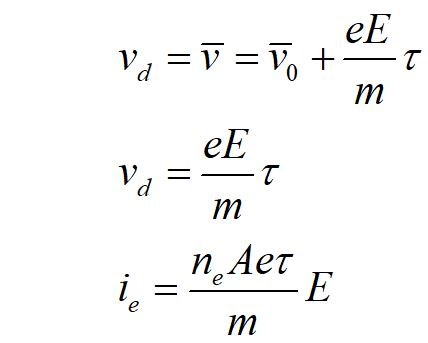The average velocity is equal to the drift speed. The time between collisions is defined as τ. We can use substitution to write the electron current in terms of the drift speed and τ.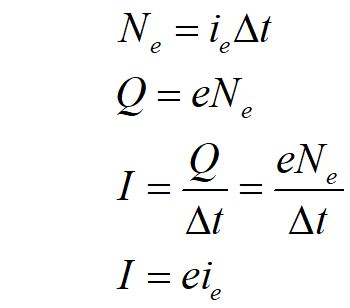## Conservation in electric current

### Electrons flowing through a wire obey conservation laws. Electrons cannot be created or destroyed as they flow through a wire or a component of an electrical circuit like a light bulb or resistor. Each electron carries its fundamental charge, so the amount of charge flowing is also conserved. Energy carried by the electrons is used up as they pass through the filament of a light bulb. Some of the energy given to the electrons by the battery or other power source is converted to thermal energy. The current is not used up. The rate of flow of electrons into the filament of a light bulb is the same as the rate of flow of electrons out of the bulb. Current is constant everywhere in a circuit, as long as there are no junctions involved.